Next: Methodologies Up: PHYS 560: Lectures During Previous: Pions

# Isospin

At the quark level we have motivated the existence of a symmetry called isospin. The world is very nearly invariant at the level of strong interactions under the continuous (unitary) transformation that mixes the up and down type quarks. Clearly, when we consider electromagnetic and weak interactions, in which the up and down quarks couple differently, this is not the case, but lets forget this at present.

Turning to the nucleons, and forgetting about weak and electromagnetic interactions, with identical spins and nearly identical masses it is a very good approximation to say that the world would be invariant under continuous, unitary transformations that mix the proton and neutron. Another way of saying this is that the strong Hamiltonian (Lagrangian) is invariant under isospin transformations.

We group the proton and the neutron together into the fundamental representation of the SU(2), and define the isospin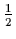nucleon field, N,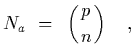(12)

i.e.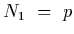and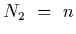. Forgetting about spin, the quantities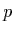and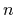are annihilation operators, we will return to this shortly. Under isospin transformations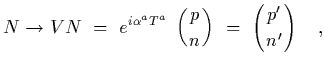(13)

where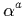are three real numbers and the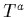are the (hermitian) generators of SU(2), related to the pauli matrices by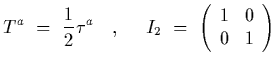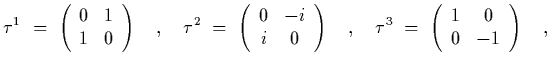(14)

where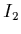is the identity matrix. We have the following nice operations of the matrices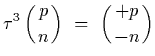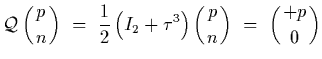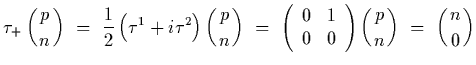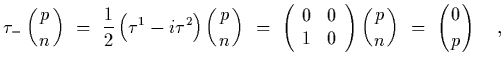(15)

where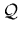is the electromagnetic charge matrix, that will be useful later, an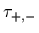are isospin raising and lowering matrices. I have written the above relations in terms of field operators, and in non-relativistic quantum mechanical notation, we define the proton state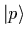and the neutron state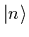as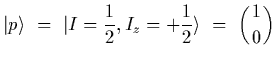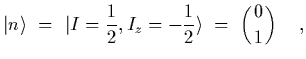(16)

where the two-component vector is in isospin space. There are a couple of relations that helps in the manipulation of these two-component objects (both in spin and isospin space)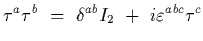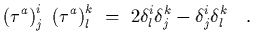(17)

So for instance, if the two-component objects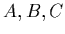,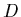are comprised of commuting (bosonic) objects, then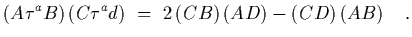(18)

Further, for the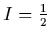system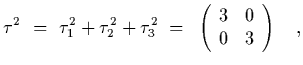(19)

which means that the eigenvalues of thesystems are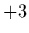.

Let us now consider the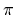's in the context of isospin. There are three different charge states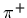,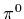and. They fit simply into a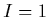multiplet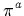, corresponding to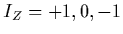respectively, with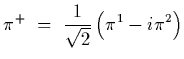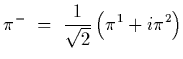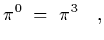(20)

where again these are understood to be field operators. The situation is a little different for these operators, compared to those associated with the nucleon field. Theoperators can annihilate or create a. We will not use this fact much, but keep it in mind.

Fo our purposes, we will only be considering the pion in the context of nucleons, i.e. for nuclear physics purposes, and so it is simpler for us to put the pions into the adjoint representation of SU(2), which is accomplished by contracting the index on thefield with the index of the pauli matrices, which defines the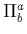field with one up and one down index,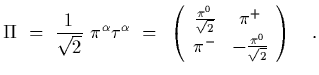(21)

In terms of states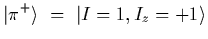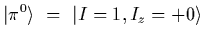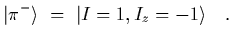(22)Next: Methodologies Up: PHYS 560: Lectures During Previous: Pions
Martin Savage
1999-10-04